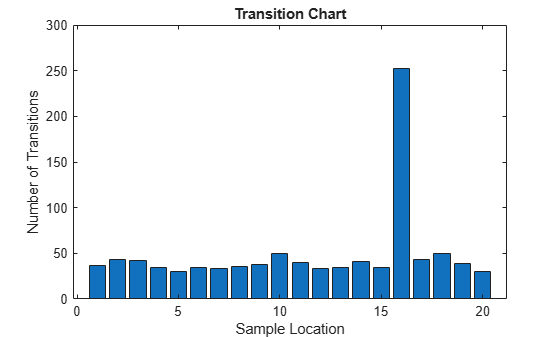# gnssBitSynchronize

## Syntax

``[syncidx,numtr] = gnssBitSynchronize(samples,n)``

## Description

example

````[syncidx,numtr] = gnssBitSynchronize(samples,n)` performs bit synchronization on the input samples `samples` with a window size of `n`, as defined in . The function searches the input samples for the maximum number of transitions from a positive to a negative value and from a negative to a positive value, and returns the bit synchronization index `syncidx` and the number of transitions corresponding to each sample location `numtr`.Use this function for global navigation satellite systems (GNSS) receivers that use code division multiple access (CDMA) schemes. These GNSS receivers include GPS, NavIC, and QZSS.```

## Examples

collapse all

Perform bit synchronization for a noisy signal generated from random bits using C/A-code. Assume perfect time and frequency synchronization.

Generate the transmit signal from random bits using C/A-code.

```n = 20; % Number of C/A-code blocks per bit numbits = 500; % Number of data bits numcachips = 1023; % Number of C/A-code chips txbits = randi([0,1],numbits,1); cacode = 1 - 2*double(repmat(gnssCACode(1,"GPS"),n*numbits,1)); txsig = cacode.*repelem(1 - 2*txbits,numcachips*n,1);```

```snrdB = -25; % Signal to noise ratio in dB rxsig = awgn(txsig,snrdB,"measured");```

Multiply the noisy signal with time synchronized reference C/A-code and integrate the samples.

```corrsamples = rxsig.*cacode; integsamples = sum(reshape(corrsamples,numcachips,[]));```

Delay the samples by a fixed offset.

```dly = 15; % Number of samples delayed bitsyncin = [zeros(dly,1);integsamples(:)];```

Perform bit synchronization on the input samples.

`[syncidx,numtr] = gnssBitSynchronize(bitsyncin,n);`

Display the value of bit synchronization index, `syncidx`. Note that it is equal to the number to samples delayed + 1.

`syncidx`
```syncidx = 16 ```

Plot the transition chart at each sample location.

```bar(numtr) xlabel("Sample Location") ylabel("Number of Transitions") title("Transition Chart")```## Input Arguments

collapse all

Input samples, specified as a real-valued column vector of length greater than or equal to `n`.

For a better estimate of the bit synchronization index, specify a large number of input samples (such as hundreds of times `n`).

Data Types: `double`

Window size used for searching the input samples, specified as a positive integer. This value represents the number of spreading code blocks per bit, or the number of samples per bit.

Data Types: `double` | `uint8`

## Output Arguments

collapse all

Bit synchronization index, returned as an integer in the range [1, `n`]. This index value represents the maximum number of transitions within the window size `n`.

The data type of the index is same as that of `n`.

Number of transitions corresponding to each sample location, returned as a column vector of length `n`.

Data Types: `double`

 GPS Enterprise Space & Missile Systems Center (SMC) - LAAFB. NAVSTAR GPS Space Segment/Navigation User Segment Interfaces. IS-GPS-200L. El Segundo, CA: SAIC (GPS SE&I), May 14, 2020. https://navcen.uscg.gov/sites/default/files/pdf/gps/IS_GPS_200L.pdf.

 Kaplan, Elliott D., and C. Hegarty, eds. Understanding GPS/GNSS: Principles and Applications. Third edition. GNSS Technology and Applications Series. Boston ; London: Artech House, 2017.